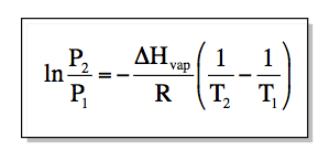Chemistry Practice Problems Clausius-Clapeyron Equation Practice Problems Solution: The vapor pressure of carbon tetrachloride is meas...

🤓 Based on our data, we think this question is relevant for Professor Wiginton's class at OLE MISS.

# Solution: The vapor pressure of carbon tetrachloride is measured as a function of temperature and the results are tabulated and shown in the table below. Determine the heat of vaporization of carbon tetrachloride from the results.Temperature  (K)Pressure  (torr)25511.326521.027536.828561.529599.0300123.8

###### Problem

The vapor pressure of carbon tetrachloride is measured as a function of temperature and the results are tabulated and shown in the table below. Determine the heat of vaporization of carbon tetrachloride from the results.

 Temperature  (K) Pressure  (torr) 255 11.3 265 21.0 275 36.8 285 61.5 295 99.0 300 123.8

###### Solution
• Heat of vaporization can be solved using the Clasius-Clapeyron equation where:• Where P is pressure (any unit will do as long as P1 and P2 have the same unit) and T is temperature in K. ΔHvap is heat of vaporization in J/mol while R is gas constant (8.314 J/mol K)

• Plugging in the values of P and T from the table provided (I'll be using 255 K and 265 K but any will do):View Complete Written Solution

Clausius-Clapeyron Equation

Clausius-Clapeyron Equation

#### Q. The vapor pressure for pure water and pure acetone is measured as a function of temperature. In each case, a graph of the log of the vapor pressure ve...

Solved • Tue Nov 27 2018 21:32:34 GMT-0500 (EST)

Clausius-Clapeyron Equation

#### Q. Propane has a normal boiling point of -42.0oC and a heat of vaporization (Hvap) of 19.04 kJ/mol. What is the vapor pressure of propane at 30.0 oC?

Solved • Tue Nov 27 2018 21:32:33 GMT-0500 (EST)

Clausius-Clapeyron Equation

#### Q. Benzene has a heat of vaporization of 30.72 kJ/mol and a normal boiling point of 80.1 oC. At what temperature does benzene boil when the external pres...

Solved • Tue Nov 27 2018 21:32:30 GMT-0500 (EST)

Clausius-Clapeyron Equation

#### Q. The vapor pressure of a substance is measured over a range of temperatures. A plot of the natural log of the vapor pressure versus the inverse of the ...

Solved • Tue Nov 27 2018 21:32:23 GMT-0500 (EST)